The vertices B and C of a Δ ABC lie on the line, , such that BC = 5 units. Then the area of this triangle, given that the point A(1, –1, 2) is.

# The vertices B and C of a Δ ABC lie on the line,, such that BC = 5 units. Then the area of this triangle, given that the point A(1, –1, 2) is.

1. A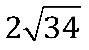2. B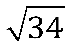3. C
6
4. D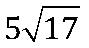Fill Out the Form for Expert Academic Guidance!l

+91

Live ClassesBooksTest SeriesSelf Learning

Verify OTP Code (required)

### Solution: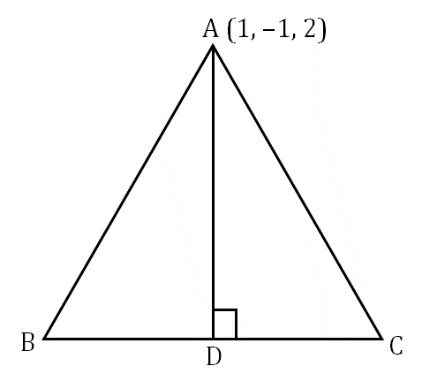‘B’, and ‘C’ lies on the line…(i)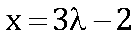,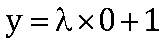,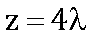y = 1
Coordinate of D{3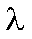–2, 1, 4}
= {3–3, 2, 4–2}
Line AD & Line 4 is perpendicular to each other
So
3( 3λ – 3) + 2 × 0 + 4( 4λ – 2) = 0
9λ – 9 + 16λ – 8 = 0
λ =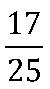Coordinate of Δ = {3λ – 2, 1, 4 λ }Area of ΔABCAD ==Area of ΔABC=unit square.

## Related content

 Area of Square Area of Isosceles Triangle Pythagoras Theorem Triangle Formula Perimeter of Triangle Formula Area Formulae Volume of Cone Formula Matrices and Determinants_mathematics Critical Points Solved Examples Type of relations_mathematics+91

Live ClassesBooksTest SeriesSelf Learning

Verify OTP Code (required)